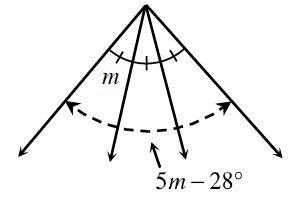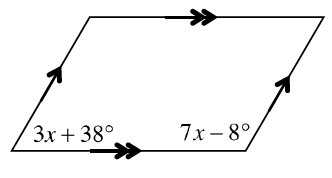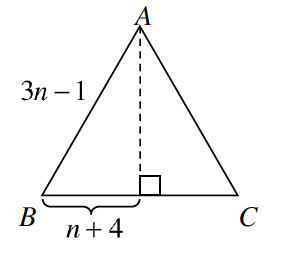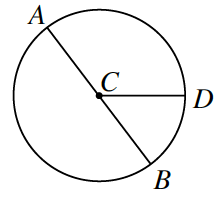### Home > CCG > Chapter 7 > Lesson 7.1.2 > Problem7-18

7-18.

Examine the geometric relationships in each of the diagrams below. For each one, write and solve an equation to find the value of the variable. Name any geometric property or conjecture that you used.

1.How do the different measures of the angle relate to each other? How can you use this to solve for $m$?

$m=14°$

1.$3x+38°+7x−8°=180°$

$x=15°$

1. $ΔABC$ below is equilateral.How do each of the sides of the triangle relate to each other if the triangle is equilateral?
How can that help you solve for $n$?

1. Point $C$ is the center of the circle. $AB=11x-1$ and $CD=3x+12$How do $AB$ and $CD$ relate to each other?